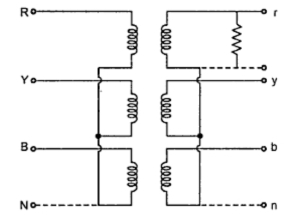### Star-Star Connection of 3-Phase Transformer

In this type of connection, both the primary and secondary windings are connected in Star as shown in the Fig. 1.This particular connection proves to be economical for small high voltage transformers as phase voltage is (1/√3) times that of line voltage, the number of turns per phase and the quantity of insulation required is minimum. The ratio of line voltages on the primary and secondary sides is the same as the transformation ratio of each transformer. It can be noted that there is a phase voltage shift of 30° between the phase voltages and line voltages on both primary and secondary side. The line voltages on both sides and the primary voltages are in phase with each other.Fig.  1

The connection of primary neutral to the neutral of generator has an add advantage that it eliminates distortion in the secondary phase voltages. If the flux in the core has sinusoidal waveform then it will give sinusoidal  waveform for the voltage. But due to characteristic of iron, a sinusoidal waveform of flux requires a third harmonic component in the exciting current. As the frequency of this component is thrice the frequency of circuit at any given constant. it will try to flow either towards or away from the neutral point in the transformer windings. With isolated neutral, the triple frequency current can not flow so the flux in the core will not be a sine wave and the voltages are distorted.
If primary neutral is connected to generator neutral the triple frequency currents get the path to solve the difficulty. The alternative way of overcoming with this difficulty is the use of tirtiary winding of low KVA rating. These windings are connected in delta and provides a circuit in which triple frequency currents can flow. Thus sinusoidal voltage on primary will give sinusoidal voltage on secondary side.
If VL1 is the line voltage on the primary side then phase voltage on primary side is given as,
If K is the turns ratio or transformation ratio then phase voltage on secondary side is given as,
...    Secondary phase voltage,

Note : Suffix 1 indicates primary side whereas suffix 2 indicates secondary side.
The line voltage on secondary side is given as,

Key point : The line voltage on secondary side is K times line voltage on primary side.

Thus in summary we can state the advantages and disadvantages of Star-Star connection as follow :
Advantages
1. Due to star connection, phase voltages is (1/√3) times the line voltage. Hence less number of turns are required. Also the stress on insulation is less. This makes the connection economical for small high voltage purposes.
2. Due to star connection, phase current is same as line current. Hence windings have to carry high currents. This makes cross section of the windings high. Thus the windings are mechanically strong and windings can bear heavy loads and short circuit.
3. There is no phase shift between the primary and secondary voltages.
4. As neutral is available, it is suitable for three phase, four wire system.
Disadvantages
1. If the load on the secondary side unbalanced then the performance of this connection is not satisfactory then the shifting  of neutral point is possible. To prevent this, star point of the primary is required to be connected to the star point of the generator.
2. Eventhough the star or neutral point of the primary is earthed, the third harmonic present in the alternator voltage may appear on the secondary side. This causes distortion in the secondary phase voltages.
Key point : Due to the disadvantages, this connection is rare in practice and used only for small high voltage transformers.

#### 1 comment:

1.where y y transformer is used in power system